International
Tables for
Crystallography
Volume C
Mathematical, physical and chemical tables
Edited by E. Prince

International Tables for Crystallography (2006). Vol. C, ch. 6.2, pp. 596-598
https://doi.org/10.1107/97809553602060000601

## Chapter 6.2. Trigonometric intensity factors

H. Lipson,a J. I. Langforda and H.-C. Hub

aSchool of Physics & Astronomy, University of Birmingham, Birmingham B15 2TT, England, and bChina Institute of Atomic Energy, PO Box 275 (18), Beijing 102413, People's Republic of China

A summary of the expressions for the intensity of diffraction as recorded by various techniques, including the fundamental constants as well as the trigonometric factors, is provided. Notes on the polarization factor, the angular-velocity factor, the Lorentz factor and special factors in the powder method are included. Some remarks are also made about the integrated reflection power ratio formulae for single-crystal slabs.

### 6.2.1. Expressions for intensity of diffraction

| top | pdf |

The expressions for the intensity of diffraction of X-rays contain several trigonometrical factors. The earlier series of International Tables (Kasper & Lonsdale, 1959, 1972) gave extensive tables of these functions, but such tables are now unnecessary, as the functions are easily computed. In fact, many crystallographers can ignore the trigonometric factors entirely, as they are built into black-box' data-processing programs. The formulae for single-crystal reflections (b) and (c) of Table 6.2.1.1in the previous edition (Lipson & Langford, 1998) list only the integrated reflection power ratio (i.e. integrated reflection) under the strong absorption case. The revised formulae given here include both the reflection power ratio and the integrated reflection power ratio for a crystal slab of finite thickness with any values of the ratio of the absorption to the diffraction cross sections and under all possible kinds of diffraction geometry.

 Table 6.2.1.1| top | pdf | Summary of formulae for integrated powers of reflection
 Absorption is neglected in both (g) and (h).
 (a) Crystal element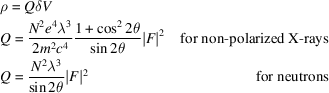(b) Reflection from a crystal slab of thickness t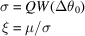1. Symmetrical Bragg geometry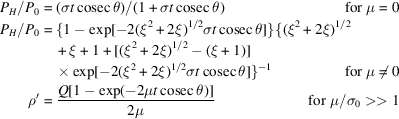2. Asymmetrical Bragg geometry, when the reflecting planes are inclined at an angleto the crystal surface, and the surface normal is in the plane of the incident and reflected beams.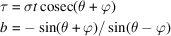angle of incidence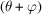and angle of emergence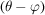to the crystal surface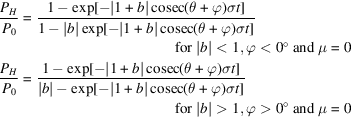Define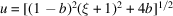and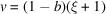: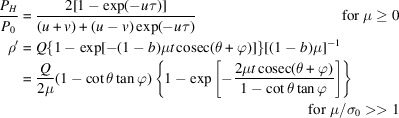(c) Transmission from a crystal slab of thickness t 1. Symmetrical Laue geometry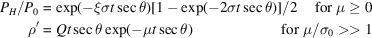2. Asymmetrical Laue geometry, when the reflecting planes are at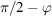to the crystal surface, with the normal in the plane of the incident and reflected beams.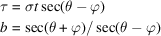angle of incidenceand angle of emergenceto the normal to the crystal surface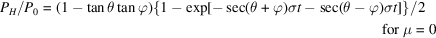Defineand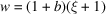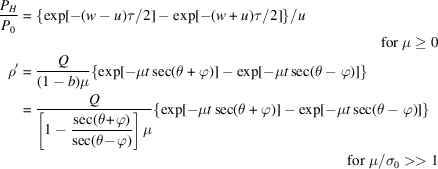(d) Powder halo: no absorption correction included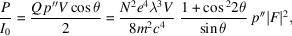where P is the diffracted power. (e) Debye–Scherrer lines on cylindrical film: no absorption correction included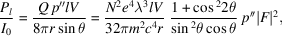where l is the length of line measured and r is the radius of the camera. Pl is the power reflected into length l. (f) Reflection from a thick block of powdered crystal of negligible transmission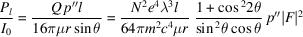(g) Transmission through block of powdered crystal of thickness t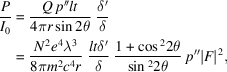where δ′, δ are the densities of the block of powder and of the crystal in bulk, respectively. (h) Rotation photograph of small crystal, volume V 1. Beam normal to axis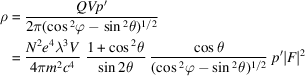2. Equi-inclination Weissenberg photograph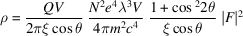Symbols
Q Integrated reflection from a crystal of unit volume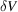Volume of crystal element
e, m Electronic charge and mass
c Speed of light
λ Wavelength of radiation
μ Linear absorption coefficient for X-rays or total attenuation coefficient for neutrons
Angle between incident and diffracted beamsIn (b) and (c), as defined; in (h), latitude of reciprocal-lattice point relative to axis of rotation
V Volume of crystal, or of irradiated part of powder sample
N Number of unit cells per unit volume
ξ In (b) and (c), as defined; in (h), radial coordinate xi used in interpreting Weissenberg photographs
I0 Energy of radiation falling normally on unit area per second
hkl Indices of reflection
F Structure factor of hkl reflection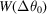Distribution function of the mosaic blocks at angular deviationfrom the average reflecting plane
σ Diffraction cross section per unit volume
σ0 Diffraction cross section per unit volume at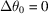b Asymmetry parameter
τ Reduced thickness of the crystal slab
PH/P0 Reflection power ratio, i.e. the ratio of the diffracted power to the incident power
ρ Integrated reflection power ratio from a crystal element
ρ′ Integrated reflection power ratio, angular integration of reflection power ratio
p Multiplicity factor for single-crystal methods
p′′ Multiplicity factor for powder methods

A conspectus of the expressions for the intensity of diffraction as recorded by various techniques, including the fundamental constants as well as the trigonometric factors, is given in Table 6.2.1.1. Details of the techniques are given elsewhere in this volume (Chapters 2.12.3) and in textbooks, such as those of Arndt & Willis (1966) for single-crystal diffractometry and Klug & Alexander (1974) for powder techniques. Notes on individual factors follow.

### 6.2.2. The polarization factor

| top | pdf |

X-rays are an electromagnetic radiation, and the amplitude with which they are scattered is proportional to the sine of the angle between the direction of the electric vector of the incident radiation and the direction of scattering. Synchrotron radiation is practically plane-polarized, with the electric vector in the plane of the ring, but the radiation from an ordinary X-ray tube is unpolarized, and it may thus be regarded as consisting of two equal parts, half with the electric vector in the plane of scattering, and half with the electric vector perpendicular to this plane. For the latter, the relevant angle is π/2, and for the former it is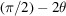. The intensity is proportional to the square of the amplitude, so that the polarization factor – really the non-polarization factor – is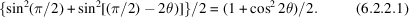If the radiation has been monochromatized' by reflection from a crystal, it will be partially polarized, and the two parts of the beam will be of unequal intensity. The intensity of reflection then depends on the angular relations between the original, the reflected, and the scattered beams, but in the commonest arrangements all three are coplanar. The polarization factor then becomes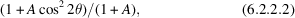where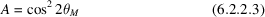andis the Bragg angle of the monochromator crystal. The expression (6.2.2.2)may be substituted for (6.2.2.1)in Table 6.2.1.1whenever appropriate.

### 6.2.3. The angular-velocity factor

| top | pdf |

In experiments where the crystal is rotated or oscillated, reflection of X-rays takes place as a reciprocal-lattice point moves through the surface of the sphere of reflection. The intensity is thus proportional to the time required for the transit of the point through the surface, and so is inversely proportional to the component of the velocity perpendicular to the surface. In most experimental arrangements – the precession camera (Buerger, 1944) is an exception – the crystals move with a constant angular velocity, and the perpendicular component of the velocity varies in an easily calculable way with the `latitude' of the reciprocal-lattice point referred to the axis of rotation. If the reciprocal-lattice point lies in the equatorial plane and the radiation is monochromatic – the most important case in practice – the angular-velocity factor is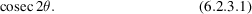If the latitude of the reciprocal-lattice point is, a somewhat more complex calculation shows that the factor becomes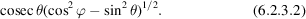For, the expression (6.2.3.2)reduces to (6.2.3.1). In some texts,is used for the co-latitude; this and various trigonometric identities can give superficially very different appearances to (6.2.3.2).

### 6.2.4. The Lorentz factor

| top | pdf |

There has been some argument over the meaning to be attached to the term Lorentz factor, probably because Lorentz did not publish his results in the ordinary way; they appear in a note added in proof to a paper on temperature effects by Debye (1914). Ordinarily, Lorentz factor is used for the trigonometric part of the angular-velocity factor, or its equivalent, if the sample is stationary. (See below).

### 6.2.5. Special factors in the powder method

| top | pdf |

In the powder method, all rays diffracted through an anglelie on the surface of a cone, and in the absence of preferred orientation the diffracted intensity is uniformly distributed over the circumference of the cone. The amount effective in blackening film, or intercepted by the receiving slit of a diffractometer, is thus inversely proportional to the circumference of the cone, and directly proportional to the fraction of the crystallites in a position to reflect. When allowance is made for these geometrical factors, it is found that for the Debye–Scherrer and diffractometer arrangements the intensity is proportional to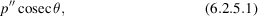where p′′ is the multiplicity factor (the number of permutations of hkl leading to the same value of). For the flat-plate front-reflection arrangement, the variation becomes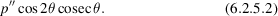Combining the polarization, angular-velocity, and special factors gives a trigonometric variation of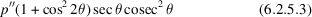for the Debye–Scherrer and diffractometer arrangements, and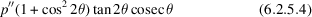for the flat-plate front-reflection arrangement.

Nowadays, angle-dispersive experiments are normally carried out by stepping the sample and detector in small angular increments, both being stationary while the intensity at each step is recorded. The Lorentz factor for a random powder sample is then of the form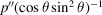. The factorarises from the fact that spherical shells of diffracted intensity in reciprocal space intersect the Ewald sphere at an angle that depends on, and the surface area of the shells increases as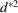, which is embodied in the factor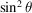. It turns out that this factor is equivalent to a combination of the polarization factor (6.2.2.1), the angular-velocity factor (6.2.3.1)and (6.2.5.1), and the form of (6.2.5.3)is thus unchanged.

### 6.2.6. Some remarks about the integrated reflection power ratio formulae for single-crystal slabs

| top | pdf |

The transfer equations for intensity may be rewritten in the form of one-dimensional power transfer equations (Hu & Fang, 1993). The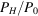in (b) and (c) for a mosaic crystal slab under symmetrical and unsymmetrical Bragg and Laue geometries are the general solutions of power transfer equations employing three dimensionless parameters b, ξ and τ. For a crystal slab with a rectangular mosaic distribution, considering multiple reflection, the integrated reflection power ratio, ρ′, can be obtained by substituting σ0 for σ in the formulae forand multiplying the result by the mosaic width. However, for crystals with other kinds of mosaic distribution, the corresponding ρ′ can be obtained only by integrating the expression for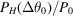over the whole range of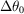. Formulae (1)–(3) listed in Table 6.3.3.1, i.e. the transmission coefficient A multiplied by Q, QA, are identical to those of (b) and (c) for the case of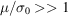, which is the integrated reflection power ratio for a crystal slab based on the kinematic approximation without consideration of multiple reflection.

The secondary extinction factor for X-ray or neutron diffraction in a mosaic crystal slab can be obtained as ρ′/(QA), in which the integrated reflection power ratio with consideration of multiple reflections can be obtained as described above.

Both the transmission power ratio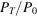and the absorption power ratio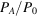can also be obtained by solving the power transfer equations. For details, see Hu (1997a,b), Werner & Arrott (1965) and Werner, Arrott, King & Kendrick (1966).

### 6.2.7. Other factors

| top | pdf |

The various expressions in Table 6.2.1.1contain |F|2, the square of the modulus of the structure factor. The relation of F to the atomic scattering factors, the atomic positional coordinates, and the temperature is treated in Chapter 6.1.

For the factors relevant for the precession method (Buerger, 1944), see Waser (1951a,b), Burbank (1952), and Grenville-Wells & Abrahams (1952). For the de Jong–Bouman method, see Bouman & de Jong (1938) and Buerger (1940). For the retigraph, see Mackay (1960).

### References

Arndt, U. W. & Willis, B. T. M. (1966). Single crystal diffractometry. Cambridge University Press.
Bouman, J. & de Jong, W. F. (1938). Die Intensitäten der Punkte einer photographierten reziproken Netzebene. Physica (Utrecht), 5, 817–832.
Buerger, M. J. (1940). The correction of X-ray diffraction intensities for Lorentz and polarization factors. Proc. Natl Acad. Sci. USA, 26, 637–642.
Buerger, M. J. (1944). The photography of the reciprocal lattice. American Society for X-ray and Electron Diffraction, Monograph No. 1.
Burbank, R. D. (1952). Upper level precession photography and the Lorentz–polarization correction. Part I. Rev. Sci. Instrum. 23, 321–327.
Debye, P. (1914). Interferenz von Röntgenstrahlen und Wärmebewegung. Ann. Phys. (Leipzig), 43, 49–95.
Grenville-Wells, H. J. & Abrahams, S. C. (1952). Upper level precession photography and the Lorentz–polarization correction. Part II. Rev. Sci. Instrum. 23, 328–331.
Hu, H.-C. (1997a). A universal treatment of X-ray and neutron diffraction in crystals. I. Theory. Acta Cryst. A53, 484–492.
Hu, H.-C. (1997b). A universal treatment of X-ray and neutron diffraction in crystals. II. Extinction. Acta Cryst. A53, 493–504.
Hu, H.-C. & Fang, Y. (1993). Neutron diffraction in flat and bent mosaic crystals for asymmetric geometry. J. Appl. Cryst. 26, 251–257.
Kasper, J. S. & Lonsdale, K. (1959). International tables for X-ray crystallography. Vol. II. Mathematical tables. Birmingham: Kynoch Press.
Kasper, J. S. & Lonsdale, K. (1972). International tables for X-ray crystallography. Vol. II. Mathematical tables. Corrected reprint. Birmingham: Kynoch Press.
Klug, H. P. & Alexander, L. E. (1974). X-ray procedures for polycrystalline and amorphous materials. New York: John Wiley.
Mackay, A. L. (1960). An axial retigraph. Acta Cryst. 13, 240–245.
Waser, J. (1951a). The Lorentz factor for the Buerger precession method. Rev. Sci. Instrum. 22, 563–566.
Waser, J. (1951b). Lorentz and polarization correction for the Buerger precession method. Rev. Sci. Instrum. 22, 567–568.
Werner, S. A. & Arrott, A. (1965). Propagation of Bragg-reflected neutrons in large mosaic crystals and the efficiency of monochromators. Phys. Rev. 140, A675–A686.
Werner, S. A., Arrott, A., King, J. S. & Kendrick, H. (1966). Propagation of Bragg-reflected neutrons in bounded mosaic crystals. J. Appl. Phys. 37, 2343–2350.